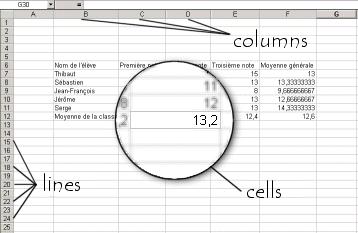# Spreadsheets - Worksheets

## The Concept of a Worksheet

Spreadsheets display data and formulas in a table form (lines and columns) called a worksheet.

A worksheet is made of lines (numbered with numbers) and of columns (numbered with letters). The intersection of a line and a column is called a cell. A cell is therefore represented by a number and a letter.

A worksheet can contain up to 65,536 lines and 256 columns, more that 17 million cells.

Each of the cells on a worksheet may contain values. These values are entered directly (numbers, text, date, etc.) or are automatically calculated by the spreadsheet. This is referred to as formulas, meaning expressions that calculate a value in function of one or more values in other cells on the worksheet.

Spreadsheets have a large number of integrated functions for math calculations, statistics, etc.

Here is an example of a worksheet that contains student's grades for which the spreadsheet automatically calculates the averages:CCM is a leading international tech website. Our content is written in collaboration with IT experts, under the direction of Jeff Pillou, founder of CCM.net. CCM reaches more than 50 million unique visitors per month and is available in 11 languages.

Learn more about the CCM team

## Related

Latest update on October 16, 2008 at 09:43 AM by Jean-François Pillou.

Recommended
TOP DOWNLOADS